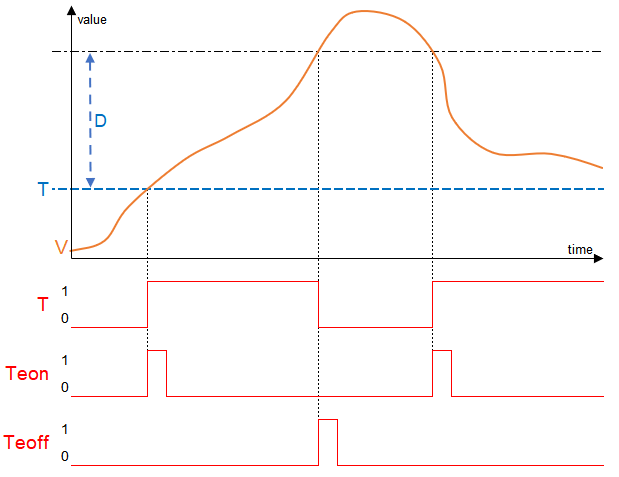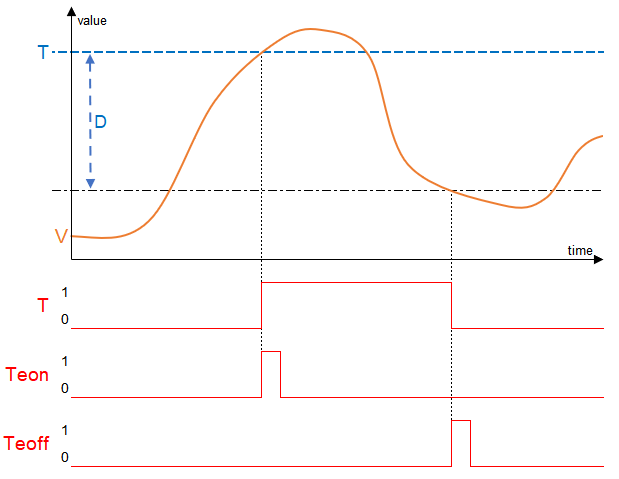# Differential Threshold Switch

The Differential Threshold Switch analyses an analogue input value using two threshold values, and switches a digital output accordingly.

The first threshold is specified with parameter (T).
The second threshold is specified with parameter (D) as a differential value relative to parameter (T).
The functionality differs, depending on whether (D) is positive or negative.

## Inputs↑

Abbreviation Summary Value Range
V Value

## Outputs↑

Abbreviation Summary Value Range
T 1 depending on the set threshold values. 0/1
Teon Pulse on rising edge 0/1
Teoff Pulse on falling edge 0/1

## Parameters↑

Abbreviation Summary Description Value Range Default Value
T Threshold 5
D Difference 2
Rem Remanence input Remanence input: If active, the function block retains its previous state after a Miniserver reboot. 0/1 0

## Function↑

Function when differential value is positive:
The second threshold value is above parameter T (T+D).
Output (T) switches on when the input value is between the threshold values.
Example: T: 5, D: 2
The output is On when the input value is between 5 and 7.
The output remains off above and below these values:Function when differential value is negative:
The second threshold value is below parameter T (T-D).
Output (T) is switched on when the input value exceeds parameter (T) and switched off when the input value drops below (T-D).
Example: T: 5, D: -2
When the input value exceeds 5, the output switches on. It remains on above this value.
If the input value drops below 3, the output switches off. Below that it remains off: